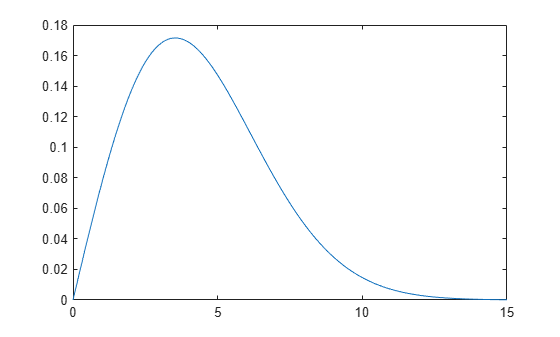# median

Median of probability distribution

## Syntax

``m = median(pd)``

## Description

example

````m = median(pd)` returns the median `m` for the probability distribution `pd````

## Examples

collapse all

Load the sample data. Create a vector containing the first column of students' exam grade data.

```load examgrades x = grades(:,1);```

Create a normal distribution object by fitting it to the data.

`pd = fitdist(x,'Normal')`
```pd = NormalDistribution Normal distribution mu = 75.0083 [73.4321, 76.5846] sigma = 8.7202 [7.7391, 9.98843] ```

Compute the median of the fitted distribution.

`m = median(pd)`
```m = 75.0083 ```

For a symmetrical distribution such as the normal distribution, the median is equal to the mean, `mu`.

Create a Weibull probability distribution object.

`pd = makedist('Weibull','A',5,'B',2)`
```pd = WeibullDistribution Weibull distribution A = 5 B = 2 ```

Compute the median of the distribution.

`m = median(pd)`
```m = 4.1628 ```

For a skewed distribution such as the Weibull distribution, the median and the mean may not be equal.

Calculate the mean of the Weibull distribution and compare it to the median.

`mean = mean(pd)`
```mean = 4.4311 ```

The mean of the distribution is greater than the median.

Plot the pdf to visualize the distribution.

```x = [0:.1:15]; pdf = pdf(pd,x); plot(x,pdf)```## Input Arguments

collapse all

Probability distribution, specified as one of the probability distribution objects in the following table.

Distribution ObjectFunction or App Used to Create Probability Distribution Object
`BetaDistribution``makedist`, `fitdist`, Distribution Fitter
`BinomialDistribution``makedist`, `fitdist`, Distribution Fitter
`BirnbaumSaundersDistribution``makedist`, `fitdist`, Distribution Fitter
`BurrDistribution``makedist`, `fitdist`, Distribution Fitter
`ExponentialDistribution``makedist`, `fitdist`, Distribution Fitter
`ExtremeValueDistribution``makedist`, `fitdist`, Distribution Fitter
`GammaDistribution``makedist`, `fitdist`, Distribution Fitter
`GeneralizedExtremeValueDistribution``makedist`, `fitdist`, Distribution Fitter
`GeneralizedParetoDistribution``makedist`, `fitdist`, Distribution Fitter
`HalfNormalDistribution``makedist`, `fitdist`, Distribution Fitter
`InverseGaussianDistribution``makedist`, `fitdist`, Distribution Fitter
`KernelDistribution``fitdist`, Distribution Fitter
`LogisticDistribution``makedist`, `fitdist`, Distribution Fitter
`LoglogisticDistribution``makedist`, `fitdist`, Distribution Fitter
`LognormalDistribution``makedist`, `fitdist`, Distribution Fitter
`LoguniformDistribution``makedist`
`MultinomialDistribution``makedist`
`NakagamiDistribution``makedist`, `fitdist`, Distribution Fitter
`NegativeBinomialDistribution``makedist`, `fitdist`, Distribution Fitter
`NormalDistribution``makedist`, `fitdist`, Distribution Fitter
`PiecewiseLinearDistribution``makedist`
`PoissonDistribution``makedist`, `fitdist`, Distribution Fitter
`RayleighDistribution``makedist`, `fitdist`, Distribution Fitter
`RicianDistribution``makedist`, `fitdist`, Distribution Fitter
`StableDistribution``makedist`, `fitdist`, Distribution Fitter
`tLocationScaleDistribution``makedist`, `fitdist`, Distribution Fitter
`TriangularDistribution``makedist`
`UniformDistribution``makedist`
`WeibullDistribution``makedist`, `fitdist`, Distribution Fitter

## Output Arguments

collapse all

Median of the probability distribution, returned as a scalar value. The value of `m` is the 50th percentile of the probability distribution.

## Version History

Introduced in R2013a